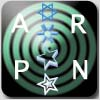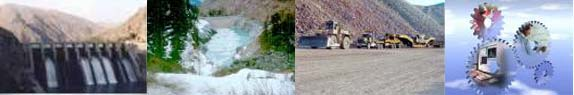ARPN Journal of Engineering and Applied Sciences                         ISSN 1819-6608Custom Search

 ARPN Journal of Engineering and Applied Sciences           September 2010 | Vol. 5  No. 9
 Title: Fast computing neural network modeling for fault diagnosis in power systems Author (s): P. Chandra Sekhar, B. V. Sanker Ram and K. S. Sarma Abstract: In this paper an approach for fault location based on online neural network is designed. The approach of learning the neural network based on the running fault values are trained for the suggested neural network. This approach result in running fault diagnosis based on the fault observation parameter based on the diagnosis tool. The approach is designed to run on running values of the distributed system so as to overcome the level of fault happening in a run time environment, which is not observed in case of the conventional neural controlling method.
 Title: Natural convection heat transfer from fin arrays-experimental and theoretical study on effect of inclination of base on heat transfer Author (s): S. V. Naidu, V. Dharma Rao, B. Govinda Rao, A. Sombabu and B. Sreenivasulu Abstract: The problem of natural convection heat transfer from fin arrays with inclination is studied experimentally and theoretically to find the effect of inclination of the base of the fin array on heat transfer rate. A numerical model is developed by taking an enclosure, which is formed by two adjacent vertical fins and horizontal base. Results obtained from this enclosure are used to predict heat transfer rate from the fin array. All the governing equations related to fluid in the enclosure, together with the heat conduction equation in both the fins are solved by using Alternate Direct Implicit method.  Numerical results are obtained for temperature along the length of the fin and in the fluid in the enclosure. The experimental studies have been also carried out on two geometric orientations viz., (a) vertical base with vertical fins (vertical fin array) and (b) horizontal base with vertical fins (horizontal fin array), with the five different inclinations like 00, 300, 450, 600, and 900. The experimental results are compared with the numerical results computed by the theoretical analysis shows the good agreement.
 Title: Numerical Simulation of Hydrodynamic Field from Pump-Turbine Runner Author (s): I. Anton and I. Sârbu Abstract: One of the fundamental hypotheses of turbomachine design is to assume that the stream surfaces in the machine bladed zones are of revolution type. This can be obtained by accepting the flow as axisymmetric in the hypothesis of incompressible and ideal fluid and by determining the hydrodynamic field constituted by the stream lines and equipotential ones, respectively, in the meridian plane. In this paper is developed a computational model of the hydrodynamic field in the meridian plane by the boundary element technique. The results are obtained by solving a boundary-limit conditions problem for Stokes’ equation for the j velocity potential. From the connection between the functions j and y is determined the stream function y and the velocity field along stream lines. The proposed computational model is applied to a pump-turbine runner and the numerical results are compared to those obtained by the finite element method.

 arpnjournals.com                                                       Publishing Policy                                                  Review Process                                           Code of Ethics